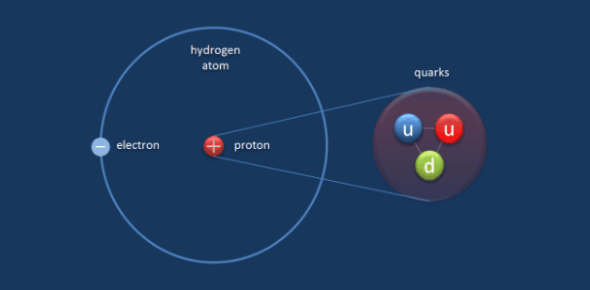# Atomic Quiz: Protons, Neutrons, And Electrons

10 Questions | Attempts: 12126SettingsAtoms are the basic building blocks of ordinary matter. Play this informative atomic quiz about proton, neutrons, and electrons to gauge your chemistry knowledge. Atoms can join together and form molecules, which also join together to create the object that we can see around us. Atoms are composed of particles called protons, electrons, and neutrons. Take up the test below and see how much you understand about them. Pick the letter you think is correct. All the best!

• 1.
Protons...
• A.

Are negatively charged

• B.

Orbit the nucleus

• C.

Are positively charged

• D.

1/2000 amu

• 2.
Galena is an ore of?
• A.

• B.

Aluminum

• C.

Nickel

• D.

Uranium

• 3.
Kryolite is an ore of?
• A.

Aluminium

• B.

Magnesium

• C.

Boron

• D.

Cobalt

• 4.
Electrons...
• A.

Orbit the nucleus and negatively charged

• B.

Are positively charged and make up the nucleus

• C.

Take up most of the mass in the atom

• D.

Are the same as the atomic number

• 5.
The nucleus
• A.

Is made up of protons and neutrons

• B.

Orbits the center proton

• C.

Is the coldest point in the atom

• D.

Surrounds the atom

• 6.
The atomic number
• A.

Tells what can and what cannot move in an atom

• B.

Orbits the center proton

• C.

States how many spoolas are in the nucleus

• D.

Is the same as the amount of protons

• 7.
AMU stands for
• A.

Absolute Mass Unit

• B.

Atomic Making Unit

• C.

Atomic Mass Unit

• D.

Abraham's Mass Unit

• 8.
If the atomic number is 9, the number of protons is
• A.

8

• B.

9

• C.

1

• D.

12

• 9.
If Uranium's atomic number is is 92 and it has 10 neutrons, how many protons does it have?
• A.

92

• B.

86

• C.

82

• D.

102

• 10.
If Aluminum has an atomic number of 13 and has 14 protons, what is its mass?
• A.

25

• B.

1

• C.

32

• D.

27

## Related TopicsBack to top
×

Wait!
Here's an interesting quiz for you.